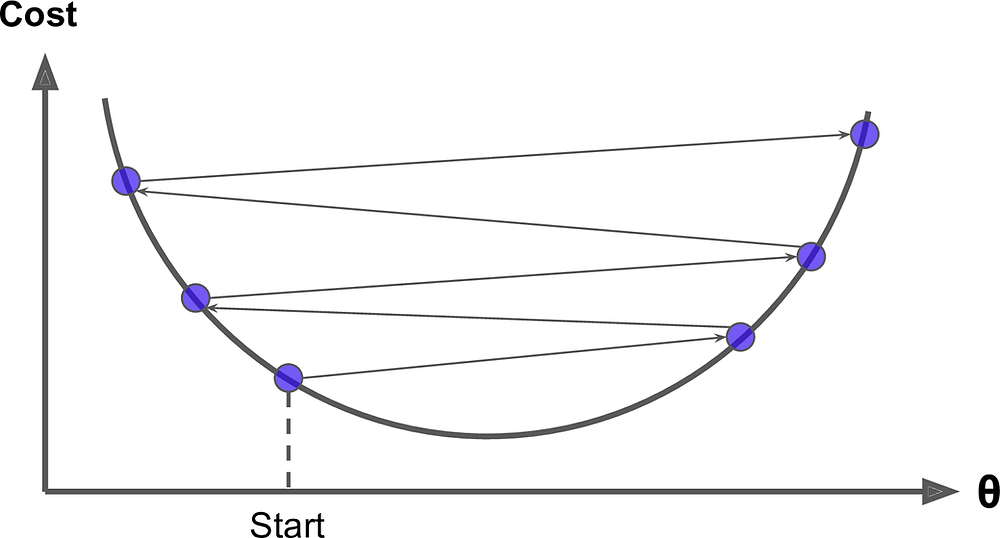Search

# Best explanation of Gradient Descent for beginners

Hello world! It's time to clear your confusion towards Gradient descent and it's workings. Today we will unfold the mystery of it.

Gradient descent is known to be a very useful algorithm and the backbone of machine learning. It has the capability of finding the best solution to a wide range of problems.

A very intuitive idea of Gradient descent is to minimize the cost function of a model by tweaking the parameters iteratively.

For example, you lost your way in a mountain and you cannot see anything because of the dense fog; your feet can feel the slope of the mountain weather you are walking upward or downwards. The best way to escape this mountain is to follow the steepest slope downhill. Gradient descent does the exact same thing. It calculates the local gradient of the error function with reference to the parameter theta (θ) or many people call it w(weights) too, and it travels in the direction where the gradient is descending. When we reach the surface i.e the gradient is zero, we reached the minimum error!

Initially, the thera(θ) are randomly assigned and then an iterative process of finding the least gradient helps us improve gradually. By taking one small step at a time, each step of it helps us decrease the cost function (in this case I am decreasing MSE) until the algorithm converges to a minima.A very important factor of gradient descent is the size of steps taken while descending downwards. It is determined by the learning rate hyperparameter. In case of a small learning rate, the algorithm has to go through plenty of iterations to converge, which is going to take a long time.On the other hand, if the learning rate is too high, you might surpass the minima and hop across to the other end of the valley probably in the higher value then before. This can force the algorithm to diverge instead of converging and failing to find a good solution irrespective of the iterations.And lastly, Not all functions look like a proper and nice bowl. There may be holes, ridges, plateaus, and all sorts of irregular terrains, making convergence to the minimum very difficult.

The below-displayed figure shows the two main challenges with Gradient Descent:

if the random initialization starts the algorithm on the left, then it will converge to a local minimum, which is not as good as the global minimum. If it starts on the right, then it will take a very long time to cross the plateau, and if you stop too early you will never reach the global minimum.Fortunately, the MSE cost function for a Linear Regression model happens to be a convex function, which means that if you pick any two points on the curve, the line segment joining them never crosses the curve.